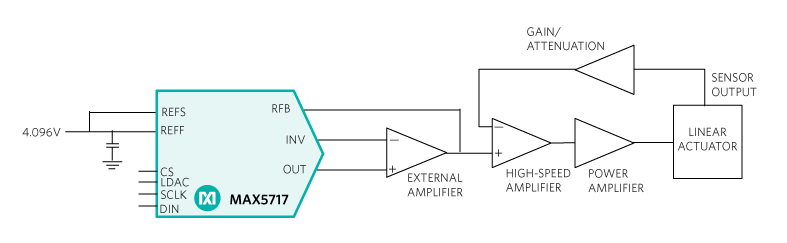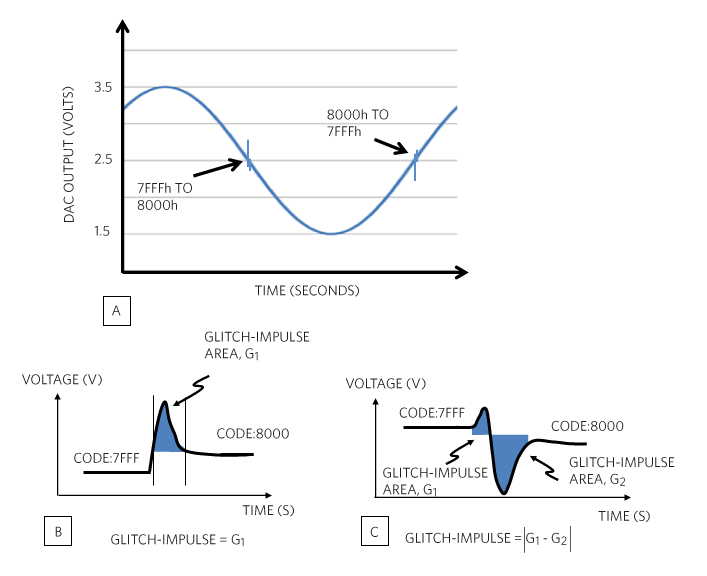# Precisely Control a Linear Actuator with a DAC

### 要約

Effective control of industrial actuators requires precise, repeatable control. Linear actuator control has a precision control loop with high resolution, monotonicity, low settling time, and low-code-to-code glitch energy. This design solution briefly discusses the issues associated with achieving precise linear actuator control and shows why linear actuators are a good option for many applications. It then reveals how to take advantage of the ultra-low settling time and glitch energy of a digital-to-analog converter (DAC).

#### Introduction

In contrast to the circular motion of an electric motor, a linear actuator creates movement in a straight line. Machine tools and industrial machinery use linear actuators in computer printers, valves and dampers, and places that require linear motion (Figure 1).

Effective control of industrial actuators requires precise, repeatable control. Effective linear actuator control has a precision control loop with high resolution, monotonicity, low-settling-time, and low-code-to-code glitch energy.Figure 1. A diagonal view of metal pinion gears on toothed bars with high-precision linear toothed wheels.

This design is challenging for designers, as precise actuators require high-precision digital-to-analog-converters (DACs) that initiate the linear movement. Often linear actuators are mechanical and too slow for the application while discrete actuators are not precise enough.

The solution for high-precision linear actuator control in many applications lies with machine tools. Industrial machinery must have precise, low-glitch, and fast DACs which create a high-precision, control system for computer printers, in valves and dampers, and in some places, in applications that require linear motion.

This design solution briefly discusses the issues associated with achieving precise linear actuator control and shows why linear actuators are a good option for many applications. It then introduces precision DACs and reveals how to take advantage of their monotonicity, ultra-low settling time, and glitch energy.

#### Linear Motion Control

Precision motion-control applications utilize high-precision control loops such as linear actuator control. Figure 2 shows a block diagram of a DAC-driven linear actuator circuit.Figure 2. Precision DAC-driven linear actuator provides precise positions during the actuator action.

In Figure 2, the high-speed DAC drives the linear actuator through the driver amplifiers. The precision 16-bit DAC sends an analog output voltage that can vary for a full-scale actuator change or for minute increases or decreases in motion.

#### DAC Monotonicity

DAC monotonicity means that an increase (or decrease) in the digital code input will always produce no change or an increase (or decrease) in the analog output.

For a linear actuator, a non-monotonic DAC would cause the actuator to shorten with an expected lengthening event. With a feedback loop, this error would cause an oscillation occurrence and the electrics in the actuator will attempt to correct the error.

If the monotonicity specification is not available in the DAC datasheet, the differential nonlinearity (DNL) specification implies the DAC’s monotonicity capability.

The DNL error is the difference between an analog actual step width and the ideal analog value of one LSB. For an ideal DAC, in which the DNL is equal to 0 LSB, each digital step equals 1 LSB. 1 LSB equals VFS /2N, where VFS is the DAC’s full-scale range and N is the DAC’s resolution. If the actual DAC step from code to code is less than 1 LSB, the DNL is negative.

A DNL error of less than -1 LSB indicates a transition value that is directly opposite of the expected transition, creating a missing code or an instance where the analog decreases with an increase in the digital input code. In other words, a DNL error that is less than -1 LSB implies that the DAC is non-monotonic.

In Figure 3, the DNL graph for a DAC displays the digital input code on the X-axis and the analog output (voltage or current) on the Y-axis.Figure 3. In this illustration, the DAC DNL determines the monotonicity of the converter, rendering this DAC to be a monotonic device.

A DNL less than -1 LSB signifies a non-monotonic DAC. For this figure, the analog output increases with increasing digital code, even when the DNL error is -½ LSB. In Figure 3, the DAC is monotonic.

#### DAC Settling-Time and Conversion Speed

Output settling time is the maximum amount of time it takes for the output of a DAC to settle to a specified level from a zero output to full-scale. In this circuit, the DAC settling time defines the speed of the actuator.

The settling time measurement starts at the falling edge of LDAC (Figure 4).Figure 4. A typical 750ns settling time of a 16-bit DAC.

Figure 4 shows an example of the settling time of an actual DAC. It is useful to note that the characteristics of the analog output signal as it spans from zero to full scale exhibits a first-order lowpass filter. The 750ns (typical) DAC settling time occurs as the signal is stable within a band of ±1 LSB around the ideal final analog voltage. Multiplying the settling time by two determines the DAC’s bandwidth—equaling 667kHz.

#### DAC Glitch Energy

Ideally DAC outputs move from one value to the next monotonically. Real circuits may have slight undershoots or overshoots. The DAC characteristics of this dynamic specification can disrupt a closed-loop linear actuator system’s operation by momentarily outputting erroneous voltages. The over or undershooting that occurs with code-to-code transitions quantifies the glitch impulse specification (Figure 5).Figure 5. (A) DAC glitch energy occurs when the digital major carrier changes in opposition to the remainder of the DAC’s codes. (B) String DACs produce a single lobe of glitch energy. (C) R-2R DACs produce a double lobe.

Figure 5 illustrates how DAC capacitive-coupled charge injection errors produce an overshoot and undershoot with the major code transition (when the DAC code changes from 1000… to 0111…). Internal DAC switch synchronization is not always perfect, and this produces an overshoot and/or undershoot signal at the output of the DAC.

Glitch impulses produced by string DACs produce a single-lobe glitch impulse (B) R-2R DACs (C) produce two regions of code transition error. In this situation, subtract the positive glitch impulse (G2) from the negative glitch impulse (G1).

Since glitch impulses are very fast, the units of measure that capture the area contained by the glitch is nanovolts-second (nV-s) (Figure 6).Figure 6. This R-2R DAC major carry (7FFF to 8000) output glitch is 0.05nV-s.

In Figure 6, the 20-bit DAC’s low 0.05nV-s glitch produces a small disruption to the operation of the linear actuator.

#### Actuator Precision from the DAC

The MAX5717/MAX5719 16-bit, precision, high-speed DACs predetermine the linear actuator’s precision performance with monotonic behavior, 750ns settling time, and ultra-low 0.05nV-s glitch energy (Figure 7).Figure 7. A block diagram of a 16-bit/20-bit DAC with a 3-wire SPI, QSPI™, MICROWIRE, and DSP-compatible serial interface.

The MAX5717/MAX5719 are serial-input, unbuffered, 16- and 20-bit voltage-output unipolar DACs with integrated feedback resistors that allow bipolar operation when used with an external op amplifier. In unipolar mode, the DACs reset to zero at power-up. These DACs provide low noise, tight bipolar resistor matching and high accuracy. Integrated precision-setting resistors make the DACs easy to use.

#### Conclusion

The need for an accurate linear actuator control does not have to be an afterthought. Simple solutions are often mechanical and too slow while discrete actuators lack precision. During the planning process, it is important to consider various specification advantages and apply an integrated solution. A precision, low-glitch, high-speed DAC provides a simple, effective feedback solution that brings peace of mind to the designer of linear actuator control.